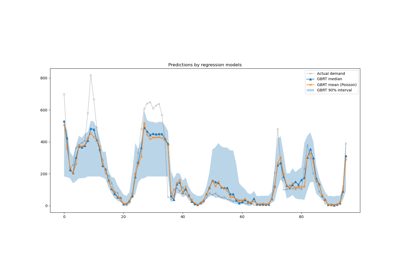# sklearn.metrics.root_mean_squared_error¶

sklearn.metrics.root_mean_squared_error(y_true, y_pred, *, sample_weight=None, multioutput='uniform_average')[source]

Root mean squared error regression loss.

Read more in the User Guide.

New in version 1.4.

Parameters:
y_truearray-like of shape (n_samples,) or (n_samples, n_outputs)

Ground truth (correct) target values.

y_predarray-like of shape (n_samples,) or (n_samples, n_outputs)

Estimated target values.

sample_weightarray-like of shape (n_samples,), default=None

Sample weights.

multioutput{‘raw_values’, ‘uniform_average’} or array-like of shape (n_outputs,), default=’uniform_average’

Defines aggregating of multiple output values. Array-like value defines weights used to average errors.

‘raw_values’ :

Returns a full set of errors in case of multioutput input.

‘uniform_average’ :

Errors of all outputs are averaged with uniform weight.

Returns:
lossfloat or ndarray of floats

A non-negative floating point value (the best value is 0.0), or an array of floating point values, one for each individual target.

Examples

>>> from sklearn.metrics import root_mean_squared_error
>>> y_true = [3, -0.5, 2, 7]
>>> y_pred = [2.5, 0.0, 2, 8]
>>> root_mean_squared_error(y_true, y_pred)
0.612...
>>> y_true = [[0.5, 1],[-1, 1],[7, -6]]
>>> y_pred = [[0, 2],[-1, 2],[8, -5]]
>>> root_mean_squared_error(y_true, y_pred)
0.822...


## Examples using sklearn.metrics.root_mean_squared_error¶Lagged features for time series forecasting

Lagged features for time series forecasting